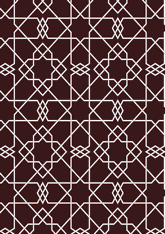# Valencia Qur'andata196/DJ1

## Geometry

• The symmetry group of the tiling is *442 (p4m).
• All the internal angles of the constituent polygons are a multiple of 15°.
• Contains one square.
• Contains two regular two-pointed star polygons with vertex angle of 60°.
• Contains two regular 8-pointed star polygons with vertex angle of 90°.
• There are five non-regular reflective tiles (including one kite).
• The tiling satisfies the interlace condition and has one finite interlace and one infinite interlace with straight cross-overs.
• The tiling is edge-to-edge.
• As drawn, contains about 423 polygons.

## References

Publications referenced:
1. Page 20 (Valencia, Spain. Qur'an) of David James. The Master scribes: Qur'ans of the 10th to 14th c, Oxford, 1992. ISBN 019727601. [james] {p240}(1199AD, 595AH)

v53